Vibroengineering PROCEDIA

Published: 15 March 2019

# Adomian’s decomposition method to modeling power functionally graded thermoelastic materials in heat transfer and thermal stress analysis

Hamdy M. Youssef1
Eman A. N. Al-Lehaibi2
1Mathematics Department, Faculty of Education, Alexandria University, Alexandria, Egypt
2Mathematics Department, Al-Lith University College, Umm Al-Qura University, Makka, Saudi Arabia
1Mechanics Department, Faculty of Engineering, Umm Al-Qura University, Makka, Saudi Arabia
Corresponding Author:
Hamdy M. Youssef
Views 86

#### Abstract

This work deals with an iteration method for numerical solving the problem of one-dimensional coupled thermoelasticity under given boundary conditions. This iteration based on the Adomian’s decomposition method. All the material properties have been considered variable on position with a power law. The numerical results have been calculated for different cases of the gradient parameter and the gradient index. The numerical results have been shown in figures. The gradient parameter and the gradient index have significant effects on the temperature increment, the strain, the stress, and the displacement.

## 1. Introduction

Recently, much attention has been devoted to the numerical methods in which do not require discretization of time-space variables or to the linearization of the non-linear equations . Adomian introduced the decomposition method for solving linear and non-linear ordinary and partial differential equations [2-4]. This method offers accurate and computable with approximately convergent solutions to linear and non-linear partial and ordinary differential equations [5-16]. Adomian got the solutions of many bio-mathematical models of viruses, bacteria, antigens, and tumor tissues . Adomian’s decomposition method (ADM) is to divide the given equation into linear and nonlinear parts of the equation [1, 13].

## 2. Mathematical idealizations of a FGM by using power law

This particular idealization for FGM modeling is well-known in the fracture mechanics science. The thickness $h$, the typical material properties $P\left(x\right)$ at any point at a distance $x$ from the reference surface has been modeled to this equation :

1

$R$ is the gradient parameter when it vanishes all the material properties are in the standard case with constant values. $n$ is the material gradient index which depends upon the design requirements .

## 3. Formulation the problem in general form

Consider an isotropic and thermo-elastic body in one-dimensional fill the region which is defined by where $h$ is the thickness of the body, and it is initially at rest and has been loaded by the harmonic thermal wave, and the surface is traction free.

The displacement components for one-dimension medium have the form :

2

The equation of motion:

3

The generalized equation of heat conduction has the form:

4
5

The constitutive relation takes the form:

6

In the above equations, $\theta =\left(T-{T}_{0}\right)$ is the temperature increment, $\rho \left(x\right)$ is the density, $\lambda \left(x\right)$ and $\mu \left(x\right)$ are Lame’s parameters, $K\left(x\right)$ is the thermal conductivity, $\gamma \left(x\right)$ is a material constant given by $\gamma \left(x\right)=\left[\text{3}\lambda \left(x\right)+\text{2}\mu \left(x\right)\right]{\alpha }_{T}\left(x\right)$, ${\alpha }_{T}\left(x\right)$ being the coefficient of linear thermal expansion, and ${C}_{E}\left(x\right)$ is the specific heat at constant strain.

## 4. Formulation of the problem by using the exponential law

Substitute from Eq. (7) into Eqs. (4)-(6), we get:

7
8
9

10
$\sigma =\left({\lambda }_{0}+2{\mu }_{0}\right){\left(1-R\frac{x}{h}\right)}^{n}e-{\gamma }_{0}{\left(1-R\frac{x}{h}\right)}^{2n}\theta .$

For simplicity, we use the non-dimensional variables (we will drop the primes) :

11

where:

thus, we obtain:

12
13
14
$\sigma ={\left(1-R\frac{x}{h}\right)}^{n}e-{\epsilon }_{1}{\left(1-R\frac{x}{h}\right)}^{2n}\theta .$

Eq. (13) has been reduced to the form:

15

The differential operator $L$ is defined as following [8, 10, 15]:

16
${L}_{xx}e\left(x,t\right)={L}_{tt}e\left(x,t\right)+{\epsilon }_{1}{\left(1-R\frac{x}{h}\right)}^{n}{L}_{xx}\theta \left(x,t\right),$
17

The appeared operators in the above equations are defined as:

18

Assuming that the inverse of the operator , ${L}_{xx}^{-1}$ exists in the forms [8, 10, 15]:

19

Thus, applying the inverse operator on both the sides of Eqs. (17) and (18), we obtain:

20
21
$\theta \left(x,t\right)=\theta \left(0,t\right)+{\frac{\partial \theta \left(x,t\right)}{\partial x}|}_{x=0}$

We decompose the functions $\theta \left(x,t\right)$ and $e\left(x,t\right)$ as following [8, 10, 15]:

22
23
24
$\sum _{k=0}^{\mathrm{\infty }}{e}_{k}\left(x,t\right)=e\left(0,t\right)+{\frac{\partial e\left(x,t\right)}{\partial x}|}_{x=0}$
25
$\sum _{k=0}^{\mathrm{\infty }}{\theta }_{k}\left(x,t\right)=\theta \left(0,t\right)+{\frac{\partial \theta \left(x,t\right)}{\partial x}|}_{x=0}$

We obtain these components by ${e}_{k}\left(x,t\right)$ and ${\theta }_{k}\left(x,t\right)$ the recursive formulas [8, 10, 15]:

26
27

The bounding plane $x=$ 0 is thermally loaded by harmonic heat and traction free as follows:

28

Moreover, the series solutions of Eq. (22) are convergent very rapidly in real physical problems as in [12, 13]. The convergence of the series has investigated by several authors in [1, 5, 8, 9, 12-15]. In an algorithmic form, the suitable value for the tolerance 10-6 :

## 6. The numerical results

The constants of the material properties were taken as follows : ${K}_{0}=$ 386 W/(mK), ${\alpha }_{0T}=$ 1.78×10-5K-1, ${C}_{0E}=$ 383.1 J/(kgK), $\eta =$ 8886.73 s/m2, ${T}_{0}=$ 293 K, 3.86×1010 N/m2, 7.76×1010 N/m2, 8954 kg/m3, ${\epsilon }_{1}=$ 0.0104443, ${\epsilon }_{2}=$ 1.60862, $\omega =\pi$, ${\theta }^{0}=$ 1.0, $h=$ 1.0.

Fig. 1θx,2.0 distribution for R= 0.0, 0.1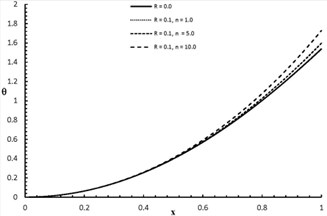Fig. 2ex,2.0 distribution when R= 0.0, 0.1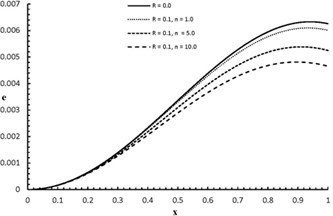Fig. 3σx,2.0 distribution when R= 0.0, 0.1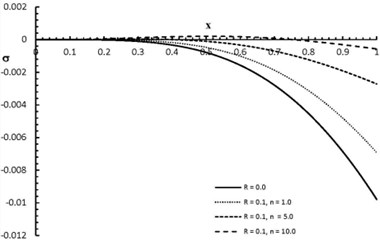Fig. 4ux,2.0 distribution when R= 0.0, 0.1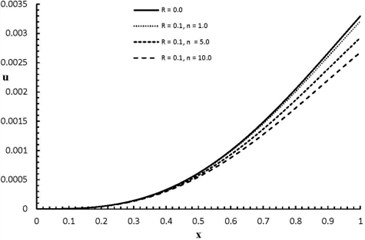Fig. 5θx,2.0 distribution when R= 0.0, 0.5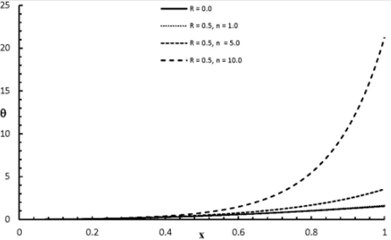Fig. 6ex,2.0 distribution when R= 0.0, 0.5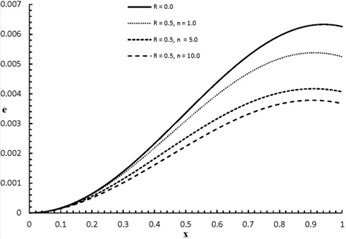Fig. 7σx,2.0 distribution when R= 0.0, 0.5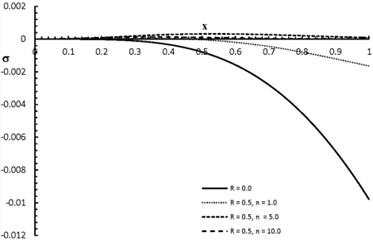Fig. 8ux,2.0 distribution when R= 0.0, 0.5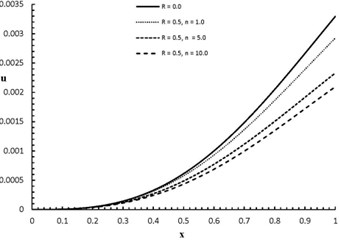## 7. Conclusions

Figs. 1-8 represent the temperature increment, the strain, the stress, and the displacement distribution with various values of the gradient parameter. $R=$ 0.0 gives the normal case of non-functionally graded material, while $R\ne \text{0.0}$ performs a functionally graded material of power law with different values$R=$ (0.1, 0.2). From the consideration in Eq. (1), $R=$ 0.1 means that the ratio ${P}_{Bot}=$ 90 % ${P}_{Top}$, and $R=$ 0.2 gives that the ratio ${P}_{Bot}=$ 80 % ${P}_{Top}$. According to the results and the figures, the parameter $R$ has significant effects on all the stat-functions $\theta =\theta \left(x,t\right)$, $e=e\left(x,t\right)$, $\sigma ={\sigma }_{xx}\left(x,t\right)$, and $u=u\left(x,t\right)$. Moreover, $n$ has been assumed with different values through the calculations ($n=$ 1, 5, 10) which give various materials’ designs. Figs.  1, 3, 5 and 7 show that when the value of the parameters $n$ and $R$ increase, the value of the temperature increment and the absolute value of the stress increase, while Figs. 2, 4, 6, and 8 show that when the value of the parameters $n$ and $R$ increases the value of the strain and the displacement decrease.